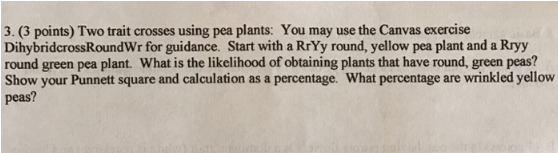# Problem: Two trait crosses using pea plants: You may use the Canvas exercise Dihybridcross RoundWr for guidance. Start with a RrYy round, yellow pea plant and a Rryy round green pea plant. What is the likelihood of obtaining plants that have round, green peas? Show your Punnett square and calculation as a percentage. What percentage are wrinkled yellow peas?

###### FREE Expert Solution

To answer this problem, write down the parent (P) generation and proceed to the Punnett square to determine its offspring.

P: RrYy (round yellow) x Rryy (round green)###### Problem Details

Two trait crosses using pea plants: You may use the Canvas exercise Dihybridcross RoundWr for guidance. Start with a RrYy round, yellow pea plant and a Rryy round green pea plant. What is the likelihood of obtaining plants that have round, green peas? Show your Punnett square and calculation as a percentage. What percentage are wrinkled yellow peas?Frequently Asked Questions

What scientific concept do you need to know in order to solve this problem?

Our tutors have indicated that to solve this problem you will need to apply the Punnett Square Probability concept. You can view video lessons to learn Punnett Square Probability. Or if you need more Punnett Square Probability practice, you can also practice Punnett Square Probability practice problems.